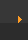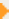## Stoichiometry: Moles to Liters

To convert between moles and liters you need to remember that one mole of a gas occupies 22.4 liters.

 Method 1 (watch video tutorial): Use the conversion factor: 1 mol gas = 22. 4L Method 2: (watch video tutorial) When going from moles to liters you multiply by 22.4. When going from liters to moles you divide by 22.4.

### Practice Problems with Key and Video Explanations

 Convert 17.5 moles Ne gas to Liters. Watch Video Solution Convert 1.8 moles Ne gas to Liters. Watch Video Solution Convert 261.5 L of CO2 gas to moles.. Watch Video Solution How many moles are in 3.7 L of H2 gas?. Watch Video Solution How many liters would 32.0 moles of Ar gas occupy?. Watch Video Solution Convert 96.8 liters of N2 gas to moles.. Watch Video Solution

### More Videos

Converting between Liters and Moles using the Factor Label Method

This is the method of choice since you can use it to convert between any units (mols to grams, molecules to mols, etc) as long as you know the conversion factor. Successful scientists use the factor label method (also called dimensional analysis).

An Alternative Method

Often students are taught to convert between liters and moles conversions by multiplying or dividing by 22.4 L/mol. This video provides information and practice on that approach.

Another example: Convert 3.7 moles of a gas to liters.

Next SectionChoose the method for converting between liters and moles based on what your instructor recommends. If you are going on in science, medicine, engineering ... you'll want to make sure you are comfortable with Method 1 (the Factor Label Method, also called Dimensional Analysis).